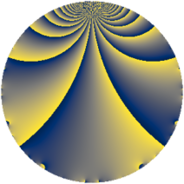# Properties

 Label 1183.2.cLevel $1183$ Weight $2$ Character orbit 1183.c Rep. character $\chi_{1183}(337,\cdot)$ Character field $\Q$ Dimension $78$ Newform subspaces $10$ Sturm bound $242$ Trace bound $3$

# Related objects

## Defining parameters

 Level: $$N$$ $$=$$ $$1183 = 7 \cdot 13^{2}$$ Weight: $$k$$ $$=$$ $$2$$ Character orbit: $$[\chi]$$ $$=$$ 1183.c (of order $$2$$ and degree $$1$$) Character conductor: $$\operatorname{cond}(\chi)$$ $$=$$ $$13$$ Character field: $$\Q$$ Newform subspaces: $$10$$ Sturm bound: $$242$$ Trace bound: $$3$$ Distinguishing $$T_p$$: $$2$$

## Dimensions

The following table gives the dimensions of various subspaces of $$M_{2}(1183, [\chi])$$.

Total New Old
Modular forms 134 78 56
Cusp forms 106 78 28
Eisenstein series 28 0 28

## Trace form

 $$78q - 80q^{4} + 86q^{9} + O(q^{10})$$ $$78q - 80q^{4} + 86q^{9} + 8q^{10} + 28q^{12} + 4q^{14} + 68q^{16} + 8q^{17} - 32q^{22} - 4q^{23} - 90q^{25} + 12q^{27} + 12q^{29} - 8q^{30} + 4q^{35} - 88q^{36} + 4q^{38} - 4q^{40} + 4q^{42} + 44q^{43} - 24q^{48} - 78q^{49} - 8q^{51} - 4q^{53} - 28q^{55} - 12q^{56} - 28q^{61} - 4q^{62} - 48q^{64} - 52q^{66} - 32q^{68} + 20q^{69} - 4q^{74} - 28q^{75} - 16q^{77} - 36q^{79} + 94q^{81} + 84q^{82} + 32q^{87} + 88q^{88} + 60q^{90} + 16q^{92} - 60q^{94} - 48q^{95} + O(q^{100})$$

## Decomposition of $$S_{2}^{\mathrm{new}}(1183, [\chi])$$ into newform subspaces

Label Dim. $$A$$ Field CM Traces $q$-expansion
$$a_2$$ $$a_3$$ $$a_5$$ $$a_7$$
1183.2.c.a $$2$$ $$9.446$$ $$\Q(\sqrt{-1})$$ None $$0$$ $$-4$$ $$0$$ $$0$$ $$q-2q^{3}+2q^{4}-3iq^{5}-iq^{7}+q^{9}+\cdots$$
1183.2.c.b $$2$$ $$9.446$$ $$\Q(\sqrt{-1})$$ None $$0$$ $$0$$ $$0$$ $$0$$ $$q+2iq^{2}-2q^{4}+3iq^{5}-iq^{7}-3q^{9}+\cdots$$
1183.2.c.c $$4$$ $$9.446$$ $$\Q(i, \sqrt{5})$$ None $$0$$ $$-6$$ $$0$$ $$0$$ $$q+(\beta _{1}-\beta _{3})q^{2}+(-1+\beta _{2})q^{3}+3\beta _{2}q^{4}+\cdots$$
1183.2.c.d $$4$$ $$9.446$$ $$\Q(\zeta_{8})$$ None $$0$$ $$0$$ $$0$$ $$0$$ $$q+\zeta_{8}^{2}q^{2}-\zeta_{8}^{3}q^{3}+(3\zeta_{8}+\zeta_{8}^{2})q^{5}+\cdots$$
1183.2.c.e $$4$$ $$9.446$$ $$\Q(\zeta_{12})$$ None $$0$$ $$4$$ $$0$$ $$0$$ $$q-\zeta_{12}^{2}q^{2}+(1+\zeta_{12}^{3})q^{3}-q^{4}-\zeta_{12}^{2}q^{5}+\cdots$$
1183.2.c.f $$6$$ $$9.446$$ 6.0.399424.1 None $$0$$ $$-4$$ $$0$$ $$0$$ $$q-\beta _{5}q^{2}+(-1-\beta _{1}-\beta _{3})q^{3}+(-1+\cdots)q^{4}+\cdots$$
1183.2.c.g $$8$$ $$9.446$$ 8.0.$$\cdots$$.1 None $$0$$ $$2$$ $$0$$ $$0$$ $$q+\beta _{1}q^{2}+\beta _{4}q^{3}+(-1+\beta _{2}+\beta _{3}+\cdots)q^{4}+\cdots$$
1183.2.c.h $$12$$ $$9.446$$ $$\mathbb{Q}[x]/(x^{12} + \cdots)$$ None $$0$$ $$-8$$ $$0$$ $$0$$ $$q+(-\beta _{8}+\beta _{10}-\beta _{11})q^{2}+(-1-\beta _{4}+\cdots)q^{3}+\cdots$$
1183.2.c.i $$12$$ $$9.446$$ 12.0.$$\cdots$$.1 None $$0$$ $$0$$ $$0$$ $$0$$ $$q+(\beta _{1}-\beta _{7})q^{2}-\beta _{2}q^{3}+(-1-\beta _{4}+\cdots)q^{4}+\cdots$$
1183.2.c.j $$24$$ $$9.446$$ None $$0$$ $$16$$ $$0$$ $$0$$

## Decomposition of $$S_{2}^{\mathrm{old}}(1183, [\chi])$$ into lower level spaces

$$S_{2}^{\mathrm{old}}(1183, [\chi]) \cong$$ $$S_{2}^{\mathrm{new}}(91, [\chi])$$$$^{\oplus 2}$$$$\oplus$$$$S_{2}^{\mathrm{new}}(169, [\chi])$$$$^{\oplus 2}$$